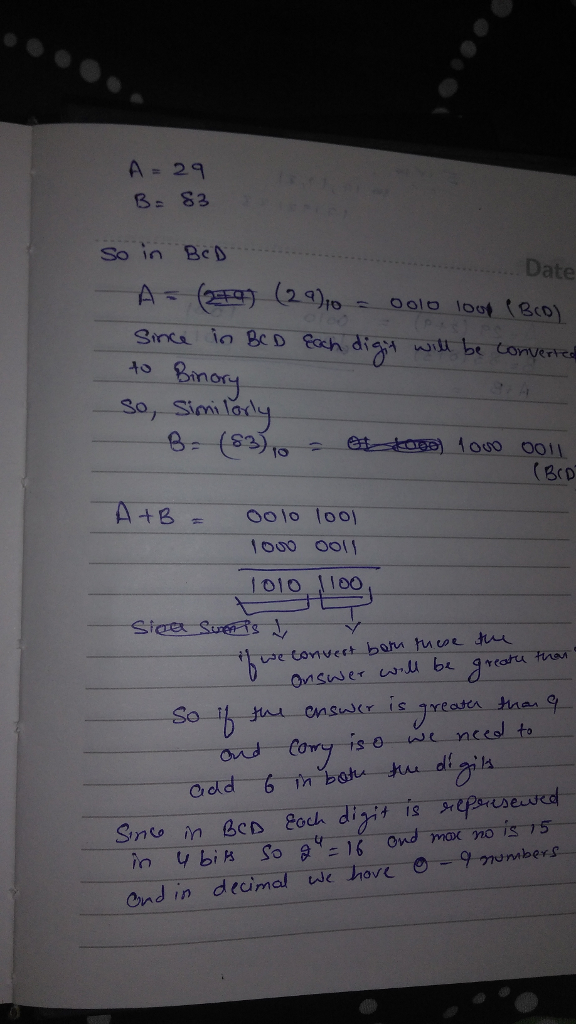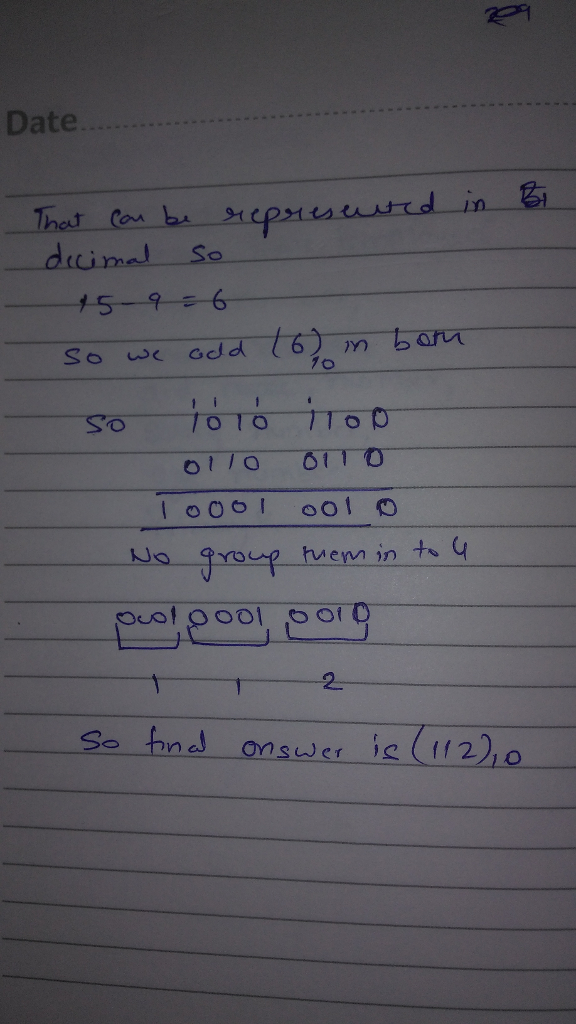# Homework Solution: Give that A= 29 and B=83 in decimal, convert to BCD representation and then perform the addition of A+…

Give that A= 29 and B=83 in decimal, convert to BCD representation and then perform the addition of A+B in while in BCD representation, show every step and all carries. Convert your answer back to decimal when done. I do rate the answer by the way